oomph::PseudoBucklingRing Class Reference

Pseudo buckling ring: Circular ring deformed by the N-th buckling mode of a thin-wall elastic ring. More...

#include <pseudo_buckling_ring.h>Inheritance diagram for oomph::PseudoBucklingRing:

## Public Member Functions

PseudoBucklingRing ()
Default constructor (empty and broken) More...

PseudoBucklingRing (const Vector< Data * > &geom_data_pt, TimeStepper *time_stepper_pt)
Constructor: 1 Lagrangian coordinate, 2 Eulerian coords. Pass buckling amplitude, ratio of of buckling amplitudes, buckling wavenumber (as a double), undeformed ring radius (all as Data) and pointer to global timestepper. More...

PseudoBucklingRing (const double &eps_buckl, const double &ampl_ratio, const unsigned n_buckl, const double &r_0, const double &T, TimeStepper *time_stepper_pt)
Constructor: 1 Lagrangian coordinate, 2 Eulerian coords. Pass buckling amplitude, ratio of of buckling amplitudes, buckling wavenumber, undeformed ring radius, period of osc and pointer to global timestepper. All geometric data is pinned by default. More...

PseudoBucklingRing (const double &eps_buckl, const double &HoR, const unsigned &n_buckl, const unsigned &imode, TimeStepper *time_stepper_pt)
Constructor: 1 Lagrangian coordinate, 2 Eulerian coords. Pass buckling amplitude, h/R, buckling wavenumbe and pointer to global timestepper. Other parameters get set up to represent oscillating ring with mode imode (1 or 2). All geometric data is pinned by default. More...

PseudoBucklingRing (const PseudoBucklingRing &node)=delete
Broken copy constructor. More...

void operator= (const PseudoBucklingRing &)=delete
Broken assignment operator. More...

~PseudoBucklingRing ()
Destructor: Clean up if necessary. More...

double eps_buckl ()
Access function for buckling amplitude. More...

double ampl_ratio ()
Access function for amplitude ratio. More...

double r_0 ()
Access function for undeformed radius. More...

double T ()
Access function for period of oscillation. More...

double n_buckl_float ()
Access function for buckling wavenumber (as float) More...

void set_eps_buckl (const double &eps_buckl)
Set buckling amplitude. More...

void set_ampl_ratio (const double &ampl_ratio)
Set amplitude ratio between radial and azimuthal buckling displacements. More...

void set_n_buckl (const unsigned &n_buckl)
Set buckling wavenumber. More...

void set_R_0 (const double &r_0)
Set undeformed radius of ring. More...

void set_T (const double &T)
Set period of oscillation. More...

void position (const Vector< double > &zeta, Vector< double > &r) const
Position Vector at Lagrangian coordinate zeta at present time. More...

void veloc (const Vector< double > &zeta, Vector< double > &veloc)
Parametrised velocity on object at current time: veloc = d r(zeta)/dt. More...

void accel (const Vector< double > &zeta, Vector< double > &accel)
Parametrised acceleration on object at current time: accel = d^2 r(zeta)/dt^2. More...

void position (const unsigned &t, const Vector< double > &zeta, Vector< double > &r) const
Position Vector at Lagrangian coordinate zeta at discrete previous time (t=0: present time; t>0: previous time) More...

void dposition_dt (const Vector< double > &zeta, const unsigned &j, Vector< double > &drdt)
j-th time-derivative on object at current time: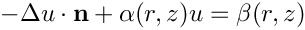. More...

unsigned ngeom_data () const
How many items of Data does the shape of the object depend on? More...

Datageom_data_pt (const unsigned &j)
Return pointer to the j-th Data item that the object's shape depends on. More...Public Member Functions inherited from oomph::GeomObject
GeomObject ()
Default constructor. More...

GeomObject (const unsigned &ndim)
Constructor: Pass dimension of geometric object (# of Eulerian coords = # of Lagrangian coords; no time history available/needed) More...

GeomObject (const unsigned &nlagrangian, const unsigned &ndim)
Constructor: pass # of Eulerian and Lagrangian coordinates. No time history available/needed. More...

GeomObject (const unsigned &nlagrangian, const unsigned &ndim, TimeStepper *time_stepper_pt)
Constructor: pass # of Eulerian and Lagrangian coordinates and pointer to time-stepper which is used to handle the position at previous timesteps and allows the evaluation of veloc/acceleration etc. in cases where the GeomData varies with time. More...

GeomObject (const GeomObject &dummy)=delete
Broken copy constructor. More...

void operator= (const GeomObject &)=delete
Broken assignment operator. More...

virtual ~GeomObject ()
(Empty) destructor More...

unsigned nlagrangian () const
Access function to # of Lagrangian coordinates. More...

unsigned ndim () const
Access function to # of Eulerian coordinates. More...

void set_nlagrangian_and_ndim (const unsigned &n_lagrangian, const unsigned &n_dim)
Set # of Lagrangian and Eulerian coordinates. More...

TimeStepper *& time_stepper_pt ()
Access function for pointer to time stepper: Null if object is not time-dependent. More...

TimeSteppertime_stepper_pt () const
Access function for pointer to time stepper: Null if object is not time-dependent. Const version. More...

virtual void position (const double &t, const Vector< double > &zeta, Vector< double > &r) const
Parametrised position on object: r(zeta). Evaluated at the continuous time value, t. More...

virtual void dposition (const Vector< double > &zeta, DenseMatrix< double > &drdzeta) const
Derivative of position Vector w.r.t. to coordinates: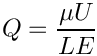= drdzeta(alpha,i). Evaluated at current time. More...

virtual void d2position (const Vector< double > &zeta, RankThreeTensor< double > &ddrdzeta) const
2nd derivative of position Vector w.r.t. to coordinates: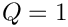= ddrdzeta(alpha,beta,i). Evaluated at current time. More...

virtual void d2position (const Vector< double > &zeta, Vector< double > &r, DenseMatrix< double > &drdzeta, RankThreeTensor< double > &ddrdzeta) const
Posn Vector and its 1st & 2nd derivatives w.r.t. to coordinates:= drdzeta(alpha,i).= ddrdzeta(alpha,beta,i). Evaluated at current time. More...

virtual void locate_zeta (const Vector< double > &zeta, GeomObject *&sub_geom_object_pt, Vector< double > &s, const bool &use_coordinate_as_initial_guess=false)
A geometric object may be composed of may sub-objects (e.g. a finite-element representation of a boundary). In order to implement sparse update functions, it is necessary to know the sub-object and local coordinate within that sub-object at a given intrinsic coordinate, zeta. Note that only one sub-object can "cover" any given intrinsic position. If the position is at an "interface" between sub-objects, either one can be returned. The default implementation merely returns, the pointer to the "entire" GeomObject and the coordinate, zeta The optional boolean flag only applies if a Newton method is used to find the value of zeta, and if true the value of the coordinate s is used as the initial guess for the method. If the flag is false (the default) a value of s=0 is used as the initial guess. More...

virtual void interpolated_zeta (const Vector< double > &s, Vector< double > &zeta) const
A geometric object may be composed of many sub-objects each with their own local coordinate. This function returns the "global" intrinsic coordinate zeta (within the compound object), at a given local coordinate s (i.e. the intrinsic coordinate of the sub-GeomObject. In simple (non-compound) GeomObjects, the local intrinsic coordinate is the global intrinsic coordinate and so the function merely returns s. To make it less likely that the default implementation is called in error (because it is not overloaded in a derived GeomObject where the default is not appropriate, we do at least check that s and zeta have the same size if called in PARANOID mode. More...

## Protected Attributes

Vector< Data * > Geom_data_pt
Vector of pointers to Data items that affects the object's shape. More...

bool Must_clean_up
Do I need to clean up? More...Protected Attributes inherited from oomph::GeomObject
unsigned NLagrangian
Number of Lagrangian (intrinsic) coordinates. More...

unsigned Ndim
Number of Eulerian coordinates. More...

TimeStepperGeom_object_time_stepper_pt

## Detailed Description

Pseudo buckling ring: Circular ring deformed by the N-th buckling mode of a thin-wall elastic ring.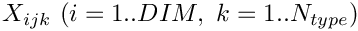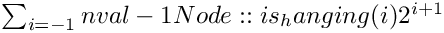where A is the ratio of the aziumuthal to the radial buckling amplitude (A=-1/N for statically buckling rings) and epsilon is the buckling amplitude.

Definition at line 55 of file pseudo_buckling_ring.h.

## ◆ PseudoBucklingRing() [1/5]

 oomph::PseudoBucklingRing::PseudoBucklingRing ( )
inline

Default constructor (empty and broken)

Definition at line 59 of file pseudo_buckling_ring.h.

## ◆ PseudoBucklingRing() [2/5]

 oomph::PseudoBucklingRing::PseudoBucklingRing ( const Vector< Data * > & geom_data_pt, TimeStepper * time_stepper_pt )
inline

Constructor: 1 Lagrangian coordinate, 2 Eulerian coords. Pass buckling amplitude, ratio of of buckling amplitudes, buckling wavenumber (as a double), undeformed ring radius (all as Data) and pointer to global timestepper.

Geom_data_pt->value(0) = Eps_buckl;
Geom_data_pt->value(1) = Ampl_ratio;
Geom_data_pt->value(2) = Double_N_buckl;
Geom_data_pt->value(3) = R_0;
Geom_data_pt->value(4) = T;
Vector< Data * > Geom_data_pt
Vector of pointers to Data items that affects the object's shape.
double T()
Access function for period of oscillation.

Definition at line 78 of file pseudo_buckling_ring.h.

References geom_data_pt(), Geom_data_pt, Must_clean_up, and oomph::Data::nvalue().

## ◆ PseudoBucklingRing() [3/5]

 oomph::PseudoBucklingRing::PseudoBucklingRing ( const double & eps_buckl, const double & ampl_ratio, const unsigned n_buckl, const double & r_0, const double & T, TimeStepper * time_stepper_pt )
inline

Constructor: 1 Lagrangian coordinate, 2 Eulerian coords. Pass buckling amplitude, ratio of of buckling amplitudes, buckling wavenumber, undeformed ring radius, period of osc and pointer to global timestepper. All geometric data is pinned by default.

Definition at line 116 of file pseudo_buckling_ring.h.

## ◆ PseudoBucklingRing() [4/5]

 oomph::PseudoBucklingRing::PseudoBucklingRing ( const double & eps_buckl, const double & HoR, const unsigned & n_buckl, const unsigned & imode, TimeStepper * time_stepper_pt )
inline

Constructor: 1 Lagrangian coordinate, 2 Eulerian coords. Pass buckling amplitude, h/R, buckling wavenumbe and pointer to global timestepper. Other parameters get set up to represent oscillating ring with mode imode (1 or 2). All geometric data is pinned by default.

Definition at line 165 of file pseudo_buckling_ring.h.

## ◆ PseudoBucklingRing() [5/5]

 oomph::PseudoBucklingRing::PseudoBucklingRing ( const PseudoBucklingRing & node )
delete

Broken copy constructor.

## ◆ ~PseudoBucklingRing()

 oomph::PseudoBucklingRing::~PseudoBucklingRing ( )
inline

Destructor: Clean up if necessary.

Definition at line 269 of file pseudo_buckling_ring.h.

References Geom_data_pt, and Must_clean_up.

## ◆ accel()

 void oomph::PseudoBucklingRing::accel ( const Vector< double > & zeta, Vector< double > & accel )
inline

Parametrised acceleration on object at current time: accel = d^2 r(zeta)/dt^2.

Definition at line 416 of file pseudo_buckling_ring.h.

Referenced by accel(), and dposition_dt().

## ◆ ampl_ratio()

 double oomph::PseudoBucklingRing::ampl_ratio ( )
inline

Access function for amplitude ratio.

Definition at line 287 of file pseudo_buckling_ring.h.

References Geom_data_pt.

Referenced by PseudoBucklingRing(), and set_ampl_ratio().

## ◆ dposition_dt()

 void oomph::PseudoBucklingRing::dposition_dt ( const Vector< double > & zeta, const unsigned & j, Vector< double > & drdt )
inlinevirtual

j-th time-derivative on object at current time:.

Reimplemented from oomph::GeomObject.

Definition at line 505 of file pseudo_buckling_ring.h.

References accel(), position(), and veloc().

## ◆ eps_buckl()

 double oomph::PseudoBucklingRing::eps_buckl ( )
inline

Access function for buckling amplitude.

Definition at line 281 of file pseudo_buckling_ring.h.

References Geom_data_pt.

Referenced by PseudoBucklingRing(), and set_eps_buckl().

## ◆ geom_data_pt()

 Data * oomph::PseudoBucklingRing::geom_data_pt ( const unsigned & j )
inlinevirtual

Return pointer to the j-th Data item that the object's shape depends on.

Reimplemented from oomph::GeomObject.

Definition at line 545 of file pseudo_buckling_ring.h.

References Geom_data_pt.

Referenced by PseudoBucklingRing().

## ◆ n_buckl_float()

 double oomph::PseudoBucklingRing::n_buckl_float ( )
inline

Access function for buckling wavenumber (as float)

Definition at line 305 of file pseudo_buckling_ring.h.

References Geom_data_pt.

## ◆ ngeom_data()

 unsigned oomph::PseudoBucklingRing::ngeom_data ( ) const
inlinevirtual

How many items of Data does the shape of the object depend on?

Reimplemented from oomph::GeomObject.

Definition at line 538 of file pseudo_buckling_ring.h.

References Geom_data_pt.

## ◆ operator=()

 void oomph::PseudoBucklingRing::operator= ( const PseudoBucklingRing & )
delete

Broken assignment operator.

## ◆ position() [1/2]

 void oomph::PseudoBucklingRing::position ( const unsigned & t, const Vector< double > & zeta, Vector< double > & r ) const
inlinevirtual

Position Vector at Lagrangian coordinate zeta at discrete previous time (t=0: present time; t>0: previous time)

Reimplemented from oomph::GeomObject.

Definition at line 452 of file pseudo_buckling_ring.h.

## ◆ position() [2/2]

 void oomph::PseudoBucklingRing::position ( const Vector< double > & zeta, Vector< double > & r ) const
inlinevirtual

Position Vector at Lagrangian coordinate zeta at present time.

Implements oomph::GeomObject.

Definition at line 344 of file pseudo_buckling_ring.h.

Referenced by dposition_dt().

## ◆ r_0()

 double oomph::PseudoBucklingRing::r_0 ( )
inline

Definition at line 293 of file pseudo_buckling_ring.h.

References Geom_data_pt.

## ◆ set_ampl_ratio()

 void oomph::PseudoBucklingRing::set_ampl_ratio ( const double & ampl_ratio )
inline

Set amplitude ratio between radial and azimuthal buckling displacements.

Definition at line 318 of file pseudo_buckling_ring.h.

References ampl_ratio(), and Geom_data_pt.

## ◆ set_eps_buckl()

 void oomph::PseudoBucklingRing::set_eps_buckl ( const double & eps_buckl )
inline

Set buckling amplitude.

Definition at line 311 of file pseudo_buckling_ring.h.

References eps_buckl(), and Geom_data_pt.

## ◆ set_n_buckl()

 void oomph::PseudoBucklingRing::set_n_buckl ( const unsigned & n_buckl )
inline

Set buckling wavenumber.

Definition at line 324 of file pseudo_buckling_ring.h.

References Geom_data_pt.

## ◆ set_R_0()

 void oomph::PseudoBucklingRing::set_R_0 ( const double & r_0 )
inline

Definition at line 330 of file pseudo_buckling_ring.h.

References Geom_data_pt, and r_0().

## ◆ set_T()

 void oomph::PseudoBucklingRing::set_T ( const double & T )
inline

Set period of oscillation.

Definition at line 336 of file pseudo_buckling_ring.h.

References Geom_data_pt, and T().

## ◆ T()

 double oomph::PseudoBucklingRing::T ( )
inline

Access function for period of oscillation.

Definition at line 299 of file pseudo_buckling_ring.h.

References Geom_data_pt.

Referenced by accel(), position(), PseudoBucklingRing(), set_T(), and veloc().

## ◆ veloc()

 void oomph::PseudoBucklingRing::veloc ( const Vector< double > & zeta, Vector< double > & veloc )
inline

Parametrised velocity on object at current time: veloc = d r(zeta)/dt.

Definition at line 381 of file pseudo_buckling_ring.h.

Referenced by dposition_dt(), and veloc().

## ◆ Geom_data_pt

 Vector oomph::PseudoBucklingRing::Geom_data_pt
protected

Vector of pointers to Data items that affects the object's shape.

Definition at line 553 of file pseudo_buckling_ring.h.

## ◆ Must_clean_up

 bool oomph::PseudoBucklingRing::Must_clean_up
protected

Do I need to clean up?

Definition at line 556 of file pseudo_buckling_ring.h.

The documentation for this class was generated from the following file: